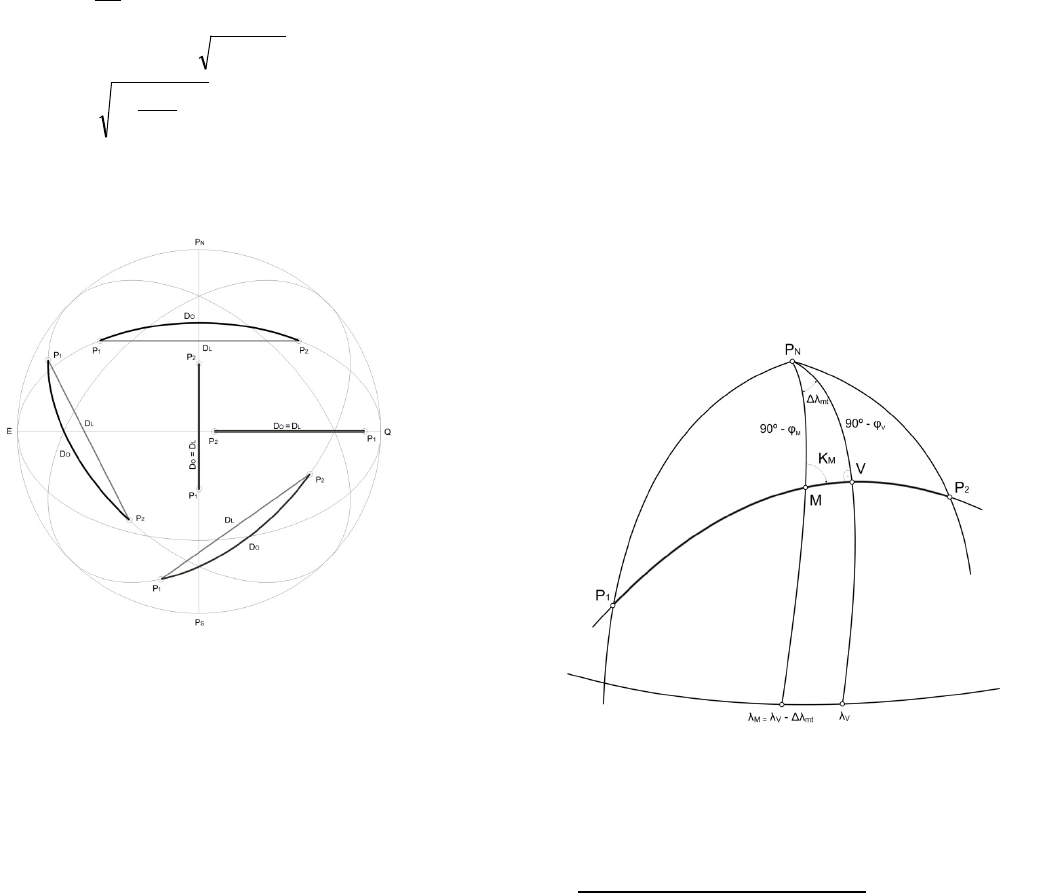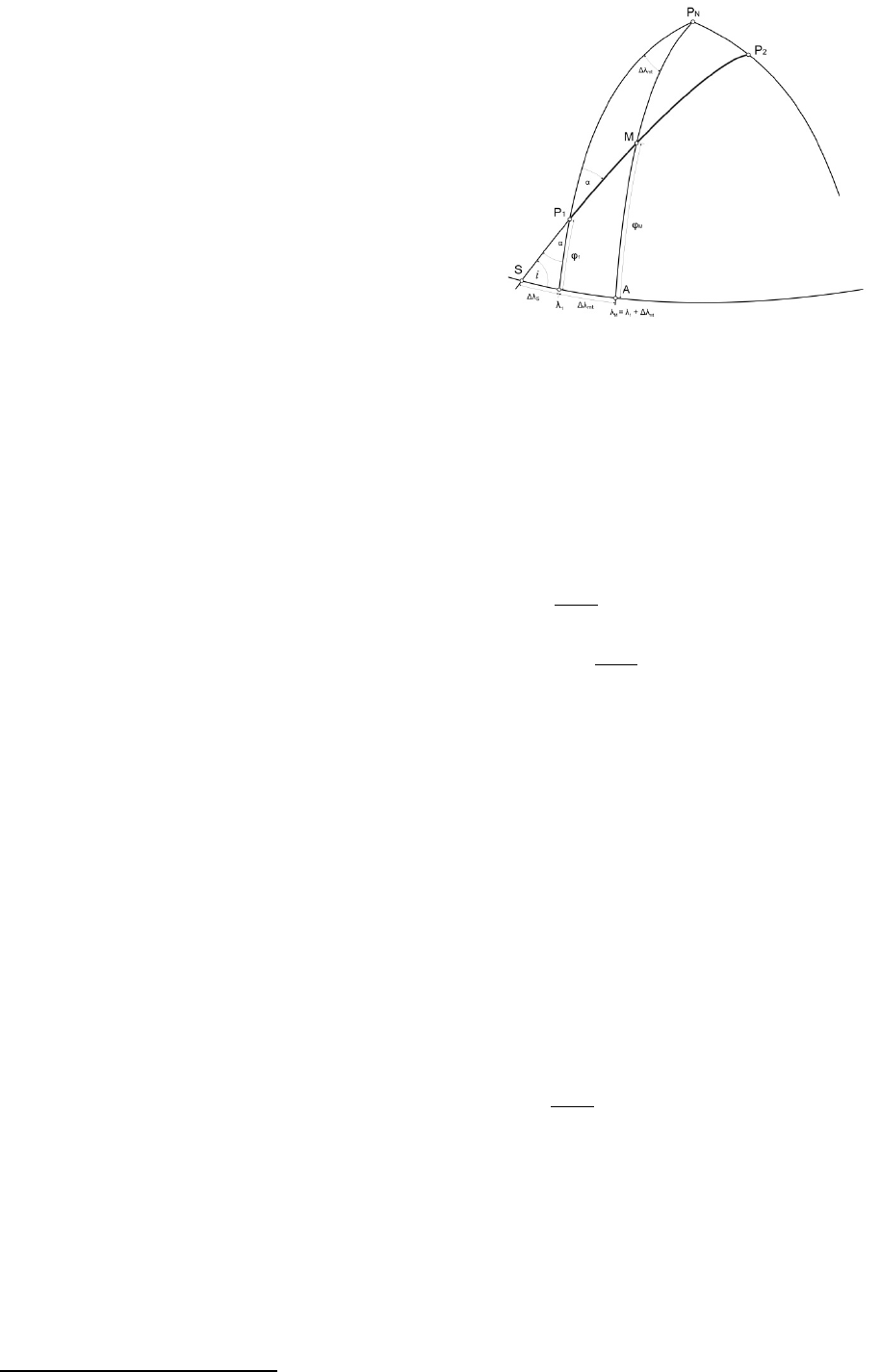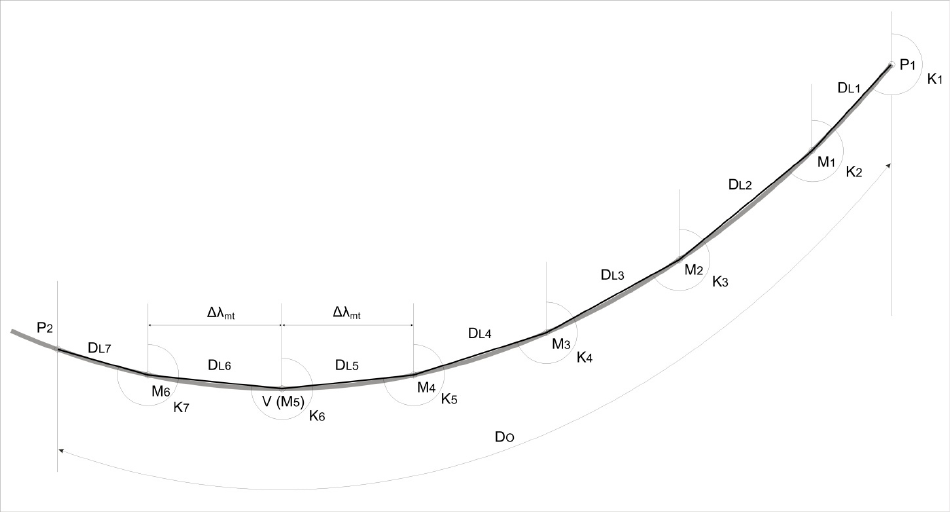International Journal
and Safety of Sea Transportation
Volume 5
Number 4
December 2011
519
1 INTRODUCTION
Navigation on the surface of the Earth is possible in
two ways: by orthodrome and loxodrome. Ortho-
drome is a minor arc of the great circle bounded by
two positions, and corresponds to their distance on a
surface of the Earth, representing also the shortest
distance between these positions on the Earth as a
sphere. The ship, travelling in orthodromic oceanic
navigation, has her bow directed towards the port of
arrival all the time. The orthodorme is the curve of a
variable course it intersects meridians at different
angles. When navigating by the orthodrome, course
should be constantly changed, which is unacceptable
from the navigational point of view. On the other
hand, loxodrome (rhumb line) intersects all meridi-
ans at the same angle, and it is more suitable in
maintaining the course. However, loxodromic path
is longer that the orthodromic one. Sailing by loxo-
drome, the bow of the ship will be directed toward
the final destination just before arrival. Due to the
mentioned facts, it is necessary to use the advantages
of both curves the shorter path of the orthodrome
and the rhumb line conformity.
Orthodrome navigation is, as mentioned, incon-
venient. Therefore, only approximation of ortho-
drome navigation can be taken into account, reduc-
ing the number of course changes to an acceptable
number always bearing in mind that if the number
of course alteration is greater, the navigation is clos-
er to the great circle. After defining elements for
course and distance determination on an orthodrome
curve, navigation between the derived points is car-
ried out in loxodromic courses.
Applying spherical trigonometry, the proposed
paper elaborates models of approximation for the or-
thodrome navigation with the secant method and the
tangent method. The secant method provides two
models of navigation. In the first model, the ortho-
drome is divided into desired waypoints interposi-
tions between which the ship sails in loxodromic
courses. The second model of the method implies
the path between two positions divided into specific
intervals of unit distances, which then define other
and loxodromic courses). In these two models, navi-
gation has been approximated with the secants of the
orthodrome curve on which the vessel sails. The
tangent method gives an approximation model by
determining the unit changes of orthodromic cours-
es, and defining the tangent on a curve, after which
are performed.
2 IMPORTANT RELATIONS BETWEEN
ORTHODROMIC AND LOXODROMIC
DISTANCES FOR THE EARTH AS A SPHERE
As described above, the rhumb line, i.e. loxodrome,
represents a constant course, spiral-shaped curve,
asymptotically approaching the Pole. The ortho-
drome represents a variable course curve, the minor
arc of the great circle between two positions. For the
Earth as a sphere, between positions P
1
and P
2
, these
two distances are equal in two situations only (Fig-
ure 1):
1 if the positions are placed on the same meridian,
then Δλ=0, Δφ≠0,
Approximation Models of Orthodromic
S. Kos & D. Brcic
University of Rijeka Faculty of Maritime Studies, Croatia
ABSTRACT: The paper deals with two different approaches to orthodromic navigation approximation, the
secant method and the tangent method. Two ways of determination of orthodromic interposition coordinates
will be presented with the secant method. In the second, tangent method unit change of orthodromic course
(ΔK) will be used.520
2 if the positions are placed on the Terrestrial Equa-
tor, then Δφ=0, Δλ≠0
In all other situations, the orthodrome distance is
always smaller, or D
O
≠ D
L
.
Maximum difference between distances D
O
and
D
L
occurs when φ
1
= φ
2
0, Δφ = 0, Δλ = 180º be-
tween P
1
and P
2
is applied. In this case, the function
extremum should be determined [Wippern, 1992]:
( )
( )
( )
( )
( )
0cos Δλ'f'
2sin Δλ f'
2πcos Δλ f
90 2cos Δλ f
<=
+=
+=
°=
ϕ
ϕ
ϕϕ
ϕϕ
ϕ
ϕ
ϕ
ϕ
For φ from to ± 90º the cosφ function is posi-
tive, so the second derivation f'
(φ)
<0. Therefore, the
function has an extremum, maximum:
( )
( )
( )
0
2
Δλ
4
1 Δλ'f'
2
sin1 Δλcos Δλ''f
Δλ
2
1
sin
02sin Δλ0f'
>=
==
=
=+=
ϕ
ϕϕ
ϕ
ϕ
ϕ
ϕ
If Δλ = 180º = π, then φ = 39º 32' 24,8''
Figure 1: Orthodrome and Loxodrome relations on Earth as a
sphere
From the completely theoretical point of view, it
follows that the strongest difference between ortho-
dromic and loxodromic distance appears if positions
P
1
and P
2
are placed on a geographic parallel of φ =
39º 32' 24,8'', and on anti-meridians, i.e. where Δλ =
180º. Then, the function value f
(φ)
amounts 2273,
5475...M [Benković et al]; this value represents the
difference between orthodromic and loxodromic
path. Maximum numerical saving of 2273,5 M in the
distance, expressed in percentage counts 37,5%
1
. In
most cases, the distance saving in percentages in
navigational practice reachs up to 10%.
In Equator/Meridian sailing, as well as heading
close to the corresponding courses, the distance sav-
ing is minimal, given that the curves are more and
more closer. In other cases, that are headings in the
090º/270º sector, particularly when sailing on the
same parallel (with appropriate distance between po-
sitions), approximating the navigation could save up
an important element of the navigation venture.
3 APPROXIMATION OF ORTHODROMIC
3.1 The first secant method – Orthodrome
interposition division
In the first secant method the problem is approached
in a way that the orthodrome is divided into interpo-
sitions, between which the vessel sails in loxodromic
courses.
Interpositions differ in their longitude every or
10º (mostly), while the division begins from the Ver-
tex of the orthodrome, under the condition that this
point is placed between the departure and arrival po-
sition. If Vertex is situated outside of the specific
positions, interpositions can be defined from the
point of departure, P
1
. In the following text, Vertex
interposition division is explained. In Figure 2. the
required relations between the elements are shown.
Figure 2: Determining the coordinates of orthodrome interposi-
tions
1
Theoretically speaking, expressed saving can reach up to 57%, but it
has no practical importance for navigation, because these are very
short paths between positions on the parallel at the near Pole (e.g.
φ=88º).521
V Vertex of the orthodrome, defined by the coor-
dinates φ
V
i λ
V
Δλ
mt
the selected difference of longitude for which
interpositions are required
M orthodrome interposition, defined by the coor-
dinates φ
M
i λ
M
The navigator selects the interposition longitude:
mtVM
λλλ =
The latitude is obtained by applying spherical
trigonometry for the right-angled triangle ΔP
N
MV
[Kos et al, 2010]:
In case that the Vertex lies outside positions P
1
and P
2
, the division begins from the point P
1
. Here,
the inclination of the orthodrome (i) should be de-
termined first. The inclination of orthodrome repre-
sents the angle at which orthodrome intersects the
Equator of the Earth, resulting in a right triangle of
the point of departure, P
1
.
)αsin (cos sin arc
αsin coscos
αsin )90( sincos
1
1
1
ϕ
ϕ
ϕ
=
=
°=
i
i
i
The longitude of the intersection, λ
S
, is defined as
follows:
S1
Δλλλ +=
S
where
tgαsintgΔ
1S
ϕλ
=
) tgαsin( tgarcΔλ
1S
ϕ
=
2
In a right-angled triangle ΔSAM, equatorial leg (Δλ
S
+ Δλ
mt
) and the angle of inclination i are known. The
following relation are a result of this triangle (Figure
3) [Kos et al, 2010]:
)90( ctg ctg)]λλ(90[ cos
MmtS
ϕ
°=+° i
MmtS
tg ctg)λλ( sin
ϕ
i=+
i tg)ΔλΔλ( sintg
mtSM
+=
ϕ
] tg)ΔλΔλ( [sin tgarc
mtSM
i+=
ϕ
2
Some of mentioned elements perhaps require additional explanation,
mathematical derivation respectively. Bearing in mind the length limi-
tation of the paper, as well as the extensive nature of the matter, the
Figure 3: The inclination of the orthodrome
3.1.1 Loxodromic intercourse and distances deter-
mination
The loxodromic courses between the positions are
calculated from the loxodromic triangle [Benković
et al, 1986]:
=
=
M
M
Δ
Δλ
tgarcK
Δ
Δλ
tgK
ϕ
ϕ
The first course (K
1
), by which the orthodrome
1
), is calculated on
the basis of Δλ, that is, the longitude difference be-
tween P
1
and the first interposition, M
1
, and the
Mercator latitudes difference between the same
points. The second course (K
2
) in M
2
is calculated
on the basis of analogic Δλ and Δφ
M
points M
1
and
M
2
, etc. The Figure 4. shows graphic determination
of loxodromic courses and distances.
Besides loxodromic courses between two interpo-
sitions, to determine the distances, one needs to
know the latitude difference between the positions,
beginning at P
1
and M
1
, then M
1
and M
2
and so on to
the point of arrival P
2
;
cosK
Δ
D
L
ϕ
=522
Figure 4: Orthodromic navigation approximation by the interposition division The first secant method
3.2 The second secant method – Division of the
orthodrome in unit distance intervals
This method is based on the theoretical assumption
that the orthodrome, which passes through two posi-
tions on the surface of the Earth as a sphere, is com-
posed of an infinite number of infinitively small
loxodromes [Kos, 1996], i.e.
=
L
O
LO
ΔdD
It follows that the final greatness of the ortho-
drome passing through two positions that are suffi-
ciently distant from each other, can be replaced with
the infinitivelly small number of loxodromes, i.e.
dD
L
= dD
O
, respectively:
=
O
D
O
OO
dDD
Given that the greatness of infinitively small lox-
odrome cannot be dimensionally defined, the loxo-
drome could be defined by the approximation of the
greatness of orthodromic unit distance intervals
(dD
O
), which is then approximately equal with the
loxodromic distance (dD
L
). In this way, the incon-
venient orthodrome navigation is replaced with the
loxodrome sailing. The intention is that the course
alternations are reduced to a navigationally accepta-
ble amount. The smaller the greatness of orthodrom-
ic unit distance, the minor the error of orthodromic
approximation. However, it requires more frequent
course alternation, which is in contradiction with
practical navigation. Therefore, it is proposed as fol-
lows:
- if two positions on Earth (approximated by the
shape of the sphere) are distant one from another
30' = 30 M, the following approximation can be in-
troduced:
DL
DO = 30'
Based on the above mentioned, the concept of unit
distance interval is introduced, and it is 30', i.e.
30 M.
The process of orthodromic navigation perform-
ing is as follows:
P
1
1
, λ
1
) – departure position coordinates
P
2
2
, λ
2
) – arrival position coordinates
Orthodromic distance between P
1
and P
2
is calcu-
lated, using the equation which is derived from the
nautical positioning spherical triangle:
)Δλcos cos cossin (sin cosD
2121
1
O
ϕϕϕϕ
+=
°<<°= 180λ0 ; λλΔλ
12
Δλ represents the difference between the longi-
tudes of departure and arrival positions.
Orthodromic distance (D
O
), expressed in degrees,
is then divided into orthodromic unit distances of
0,5º from the point of departure to the point of arri-
val.